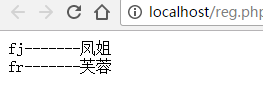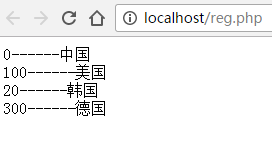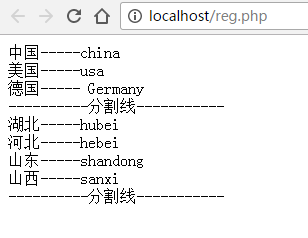• 注册

# php ​foreach遍历关联数组

foreach的基本语法

```foreach( 要循环的数组变量 as [键变量 =>] 值变量){
//循环的结构体
}```

as 是一个固定的关键字

```<?php

\$data = [
'fj' => '凤姐',
'fr' => '芙蓉',
];

foreach(\$data  as \$key => \$value){
echo \$key . '-------' . \$value . '<br />';
}

//如果我们只想读取值的话，就可以把下面的\$key => 给删除掉，读取的时候，就只读取值了。做完上面的实验，你可以打开下面的代码再实验几次。

/*
foreach(\$data  as  \$value){
echo  \$value . '<br />';
}
*/
?>```1.每次循环的时候，把下标赋值给了变量\$key，把值的变量赋值给了变量\$value

2.循环一次读一次键和值。如上例中，读完“凤姐”再读取“芙蓉”，读到最后，发现没有可以读取的数组元素后，停止循环遍历数据。

foreach还挺好学的。因此，我们可以通过foreach遍历连续的索引数组，如下例：

```<?php

\$data = array(
0 => '中国',
100 => '美国',
20 => '韩国',
300 => '德国',
);

//待会儿可以自己做做实验，循环遍历一下下面的这个数组
//\$data = array(1,2,3,4,5,6,7,8,9,10);

foreach(\$data as \$k => \$v){

echo \$k . '------' . \$v .'<br />';

}

?>``````<?php

\$data = array(

0 => array(
'中国' => 'china',
'美国' => 'usa',
'德国' => ' Germany',
),

1 => array(
'湖北' => 'hubei',
'河北' => 'hebei',
'山东' => 'shandong',
'山西' => 'sanxi',
),

);

//注：我们在使用foreach循环时，第一次循环将键为0和键为1的两个数组赋值给一个变量(\$value)。然后，再套一个循环遍历这个\$value变量，\$value中的值取出来，赋值给\$k和\$v。

foreach(\$data as \$value){

//第一次循环把国家的数组赋值给了\$value
//第二次循环把中国的省份的数组又赋值给了\$value
//因此，我在循环的时候把\$value再遍历一次

foreach(\$value as \$k => \$v){
echo \$k . '-----' . \$v .'<br />';
}

//为了看的更清晰，我在中间加上华丽丽的分割线方便你来分析

echo '----------分割线-----------<br />';

}

?>```

```<?php
\$arr=array(
'教学部'=>array(
array('李某','18','人妖'),
array('高某','20','男'),
array('张某','21','妖人'),
),
'宣传部'=>array(
array('李某','18','人妖'),
array('高某','20','男'),
array('张某','21','妖人'),
),
'财务部'=>array(
array('李某','18','人妖'),
array('高某','20','男'),
array('张某','21','妖人'),
),
);
?>```1.第一次循环的时候，将数组赋值给了\$value，然后用foreach循环\$value。将二维的子数组中的键给到\$k，值赋值给变量\$v。

2.第一次循环退出子数组的循环，执行后续代码显示分割线。

3.依此类推，第二次循环也是这样。

||
<?php \$arr=array( '教学部'=>array( array('李某','18','人妖'), array('高某','20','男'), array('张某','21','妖人'), ), '宣传部'=>array( array('李某','18','人妖'), array('高某','20','男'), array('张某','21','妖人'), ), '财务部'=>array( array('李某','18','人妖'), array('高某','20','男'), array('张某','21','妖人'), ), ); ?>

• 推荐课程
• 评论
• 问答
• 笔记
• 课件下载

foreach(\$arr as \$key => \$value){ echo \$key.'<br>'; foreach(\$value as \$values){ foreach(\$values as \$key => \$v){ echo \$v; } }

1年前    添加回复 1

Apollo

3个月前    添加回复 0

foreach(\$arr as \$k => \$v){ echo \$k.'<br>'; foreach(\$v as \$kk => \$vv){ foreach(\$vv as \$kkk => \$vvv){ echo \$vvv.'，'; } echo '<br /&

8个月前    添加回复 0

<?php \$arr=array( '教学部'=>array( array('李某','18','人妖'), array('高某','20','男'), array('张某','21','妖人'), ), '宣传部'=>array( array('李某','18','人妖'), array('

9个月前    添加回复 0

<?php \$arr=array( '教学部'=>array( array('李某','18','人妖'), array('高某','20','男'), array('张某','21','妖人'), ), '宣传部'=>array( array('李某','18','人妖'), array('

9个月前    添加回复 0

peach

10个月前    添加回复 0

<?php \$arr=array( '教学部'=>array( array('李某','18','人妖'), array('高某','20','男'), array('张某','21','妖人'), ), '宣传部'=>array( array('李某','18','人妖'),

11个月前    添加回复 0

<?php \$arr=array( '教学部'=>array( array('李某','18','人妖'), array('高某','20','男'), array('张某','21','妖人'), ), '宣传部'=>array( array('李某','18','人妖'),

11个月前    添加回复 0

′─╄Ab君

\$arr=array( '教学部'=>array( array('姓名'=>'李某','年龄'=>'18','性别'=>'人妖'), array('高某','20','男'), array('张某','21','妖人'), ), '宣传部'=>array( array('李某','18','人妖

1年前    添加回复 0

foreach(\$arr as \$key=>\$item){ echo "---------".\$key."---------<br>"; foreach(\$item as \$i){ foreach(\$i as \$key=>\$a){ echo \$a; }

1年前    添加回复 0

1年前    添加回复 0

foreach(\$arr as \$value){ foreach(\$value as \$k){ foreach(\$k as \$b){ echo \$b.'<br />'; } echo '----------分割线-----------<br />'; } }

1年前    添加回复 0

1年前    添加回复 0

foreach(\$arr as \$a => \$b){ echo \$a. "<br />"; foreach(\$b as \$c => \$d){ foreach(\$d as \$f => \$g){ echo \$g."&nbsp;&nbsp;&nbsp;&q

1年前    添加回复 0

[最新 reset 的回答]问一下，第一个循环我知道\$bum=>\$b是键值对（xx部门=>[某某人,年龄,性别）。 那第二个循环把这个\$b赋值给\$bh=>\$ren是对应哪里的？第三个循环我也懵了。。。求解答

[最新 阿神 的回答]// for 循环   // foreach 循环 foreach循环主要用于数组的遍历，它的写法相比for循环要简洁很多。foreach 语句用于循环访问集合以获取所需信息，但不应用于更改集合内容以避免产生不可预知的副作用。

[最新 lizheng13738051517 的回答]这是一种语法格式, 目的是告诉解析器 ,你的按照我这样的方式来解析  比如这个例子 foreach(\$data as \$key => \$value) 就是说 你的按照键值对的方式去解析

[最新 数据分析师 的回答]\$key=>\$value这句话什么意思，求解释？-PHP中文网问答-\$key=>\$value这句话什么意思，求解释？-PHP中文网问答围观一下哦，学习一下。

foreach( 要循环的数组变量 as [键变量 =>] 值变量)，键变量是可选项

1个月前 0

1个月前 0

<?php \$arr=array( '教学部'=>array( array('李某','18','人妖'), array('高某','20','男'), array('张某','21','妖人'), ), '宣传部'=>array( array('李某','18','人妖'), array('高某','20','男'), array('张某','21','妖人'), ), '财务部'=>array( array('李某','18','人妖'), array('高某','20','男'), array('张某','21','妖人'), ), ); foreach(\$arr as \$dept){ foreach(\$dept as \$name){ foreach(\$name as \$k=>\$people){ if(\$k==0){ \$k="姓名"; echo \$k."--------".\$people."<br>"; } if(\$k==1){ \$k="年龄"; echo \$k."--------".\$people."<br>"; } if(\$k==2){ \$k="性别"; echo \$k."--------".\$people."<br>"; } } echo "-------分割线--------"."<br>"; } } ?>

1个月前 0

rock

<?php \$zuoye = [ '教学部' => [ ['李某','18','人妖'], ['高某','18','男'], ['张某','18','女'] ], '宣传部' => [ ['李某','18','人妖'], ['高某','18','男'], ['张某','18','女'] ], '财务部' => [ ['李某','18','人妖'], ['高某','18','男'], ['张某','18','女'] ], ]; foreach (\$zuoye as \$value) { # code... foreach (\$value as \$valueer) { # code... foreach (\$valueer as \$key => \$valuesan) { # code... echo \$key.'---------'.\$valuesan.'<br />'; } } echo '<hr />'; }; ?>

4个月前 0

<?php \$arr=array( '教学部'=>array( array('李某','18','人妖'), array('高某','20','男'), array('张某','21','妖人'), ), '宣传部'=>array( array('李某','18','人妖'), array('高某','20','男'), array('张某','21','妖人'), ), '财务部'=>array( array('李某','18','人妖'), array('高某','20','男'), array('张某','21','妖人'), ), ); foreach(\$arr as \$key=>\$value){ echo '<br/>'.\$key; echo '</br>'.'------------------------------'; foreach(\$value as \$dier){ echo'</br>'; foreach(\$dier as \$disan){ echo \$disan.'----'; } } } ?>

5个月前 0

<?php \$arr=array( '教学部'=>array( array('李某','18','人妖'), array('高某','20','男'), array('张某','21','妖人'), ), '宣传部'=>array( array('李某','18','人妖'), array('高某','20','男'), array('张某','21','妖人'), ), '财务部'=>array( array('李某','18','人妖'), array('高某','20','男'), array('张某','21','妖人'), ), ); foreach(\$arr as \$key=>\$value){ echo \$key; foreach (\$value as \$k => \$v) { echo "<br>"; foreach (\$v as \$a=>\$b) { echo \$b."-"; } } echo "<br>-------------------------------------------<br>"; } ?>

7个月前 0

foreach(\$arr as \$k => \$v){ echo \$k.'<br>'; foreach(\$v as \$kk => \$vv){ foreach(\$vv as \$kkk => \$vvv){ echo \$vvv.'，'; } echo '<br />'; } echo '----------分割线-----------<br />'; }

8个月前 0

\$arr[财务部]=['石某','60','老人']; unset(\$arr[宣传部]); \$arr[教学部]='女'; foreach(\$arr as \$key=>\$value){ echo \$key.'<br/>'; foreach(\$value as \$k=>\$v){ foreach(\$v as \$a=>\$b){ echo \$b; } echo '<br/>'; } echo '------分割线------'.'<br/>'; } echo '</table>';

8个月前 0

foreach(\$arr as \$bum => \$b){ echo \$bum.'<br />'; foreach(\$b as \$bh => \$ren){ echo \$bh.'--'; foreach(\$ren as \$xx){ echo '-'.\$xx; } echo '<br />' ; } }

8个月前 0

<?php \$arr=array( '教学部'=>array( array('李某','18','人妖'), array('高某','20','男'), array('张某','21','妖人'), ), '宣传部'=>array( array('李某','18','人妖'), array('高某','20','男'), array('张某','21','妖人'), ), '财务部'=>array( array('李某','18','人妖'), array('高某','20','男'), array('张某','21','妖人'), ), ); foreach(\$arr as \$key => \$value){ echo "<h2>".\$key."</h2></br>"; foreach(\$value as \$k => \$v){ for(\$i=0;\$i<count(\$v);\$i++){ echo \$v[\$i]." "; } echo "</br>"; } } ?>

9个月前 0

<?php \$arr=array( '教学部'=>array( array('李某','18','人妖'), array('高某','20','男'), array('张某','21','妖人'), ), '宣传部'=>array( array('李某','18','人妖'), array('高某','20','男'), array('张某','21','妖人'), ), '财务部'=>array( array('李某','18','人妖'), array('高某','20','男'), array('张某','21','妖人'), ), ); foreach(\$arr as \$key => \$value){ echo "<h2>".\$key."</h2></br>"; foreach(\$value as \$k => \$v){ for(\$i=0;\$i<count(\$v);\$i++){ echo \$v[\$i]." "; } echo "</br>"; } } ?>

9个月前 0

peach

foreach( \$arr as \$y =>\$v){ foreach(\$v as \$k ){ echo '<br>'; foreach(\$k as \$a){echo \$a; }; }; echo '<br>'.'---------'.\$y.'分割线----------'.'<br>'; };

10个月前 0

waiting for.

<?php \$arr=array( '教学部'=>array( array('李某','18','人妖'), array('高某','20','男'), array('张某','21','妖人'), ), '宣传部'=>array( array('李某','18','人妖'), array('高某','20','男'), array('张某','21','妖人'), ), '财务部'=>array( array('李某','18','人妖'), array('高某','20','男'), array('张某','21','妖人'), ), ); foreach(\$arr as \$k=>\$value){ echo \$k.'<br/>'; foreach(\$value as \$k2=>\$v){ echo '<br/>'; foreach(\$v as \$k3=>\$v3){ echo \$k3.'-----'.\$v3.'<br/>'; } } echo '---------分割线-----------<br/>'; } ?>

11个月前 0

No.1

1年前 0

fighting&it'sme

1年前 0